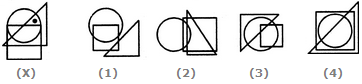# Non Verbal Reasoning - Dot Situation - Discussion

### Discussion :: Dot Situation - Section 1 (Q.No.3)

From amongst the figures marked (1), (2), (3) and (4), select the figure which satisfies the same conditions of placement of the dots as in figure (X).

3.

Select the figure which satisfies the same conditions of placement of the dots as in Figure-X.[A]. 1 [B]. 2 [C]. 3 [D]. 4

Explanation:

In fig. (X), the dot is contained in the region common to the triangle and the circle only. Out of the four alternatives, only fig. (3) contains a region common to the triangle and the circle only.

 Tinsy said: (Jul 23, 2011) I can't understand.

 Abdullah said: (Jul 25, 2013) There is option D as well wherein triangle and circle have some space common.

 A.K. Verma said: (Aug 29, 2014) There is option D is correct.

 Holly Jonson said: (Feb 6, 2016) In reply to @A.K Verma and Abdullah: The reason why D is not correct is because although the circle and triangle do overlap in answer D, the square overlaps with them (in answer D) too. But, in figure X, the dot is not going through the square therefore, that makes D incorrect. You need to find the only answer where the dot would be going through the triangle and circle while simultaneously not going through the square. The only answer that does this is C.

 Bandana said: (Jan 17, 2017) I am unable to understand this. Even in c the square overlap both triangle and square!

 Sufi said: (Mar 15, 2017) Here, we have to search for the comman portion.

 Krunal said: (Jun 30, 2017) What is the best technique to solve this type of questions? I even didn't know where to start. Is all are taking common part of diagrams of the doted part?

 Utsav Desai said: (Oct 31, 2017) In option D Circle and Triangle have common space but the whole is included in Square. In option C also Circle and Triangle have common space but Square is not included in it. Hence option C is correct.